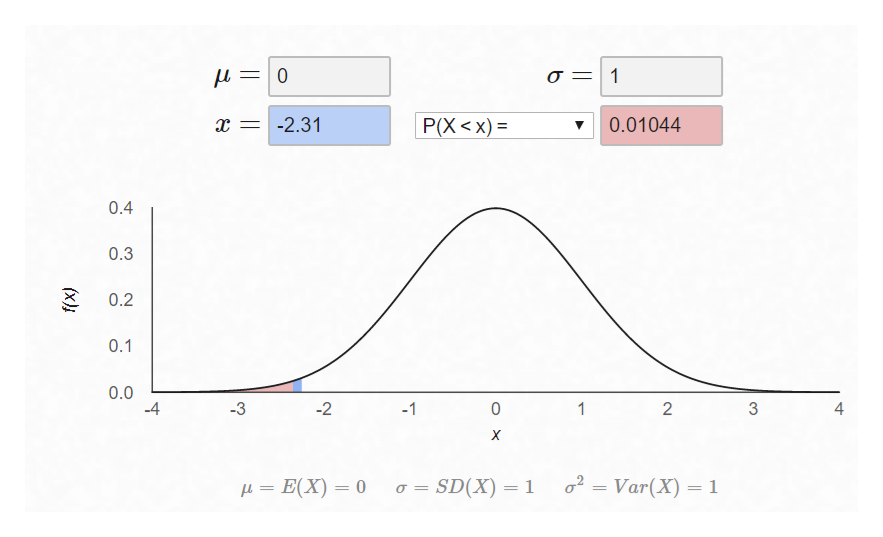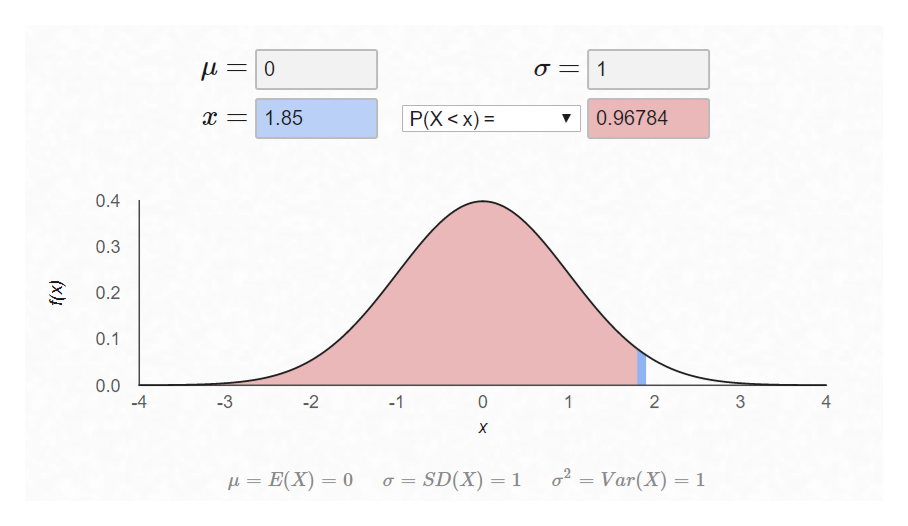# Find the percentage of data points that lie between -2.31 and 1.85.

Question

Find the percentage of data points that lie between -2.31 and 1.85.

check_circleExpert Solution
Step 1

Assuming the data follows standard normal distribution with mean 0 and standard Deviation 1
Finding the p-value at z = -2.31 = 0.010444 (From Excel = NORM.S.DIST(-2.31,TRUE)help_outlineImage TranscriptioncloseL0 -2.31 Р(X <х) %3 0.01044 0.4 0.3 0.2 0.1 0.0 -4 2 2 -3 -1 0 1 X 2 o SD(X) 1 Var(X) 1 E(X) 0 4 (x) fullscreen
Step 2

Finding the p-value at z = 1.85 = 0.967843 (From Excel = NORM.S.DIST(1.85,TRUE)help_outlineImage Transcriptionclose1 0 0.96784 1.85 Р(X <х) - 0.4 0.3 0.2 0.1 0.0 4 2 3 -1 1 2 2Var(X) 1 = E(X) = 0 a SD(X) 1 OX (x) fullscreen
Step 3

Assuming the data follows standard normal distribution with mean 0 and standard Deviation 1
Finding the p-value at z = -2.31 = 0.010444 (From Excel = NORM.S.DIST(-2.31,TR...

### Want to see the full answer?

See Solution

#### Want to see this answer and more?

Solutions are written by subject experts who are available 24/7. Questions are typically answered within 1 hour*

See Solution
*Response times may vary by subject and question
Tagged in

### Other